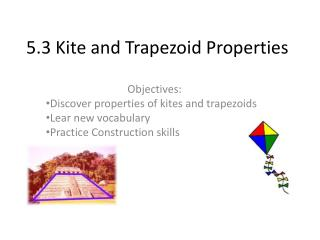# 5.3 Kite and Trapezoid Properties - PowerPoint PPT PresentationDownload Presentation5.3 Kite and Trapezoid Properties

5.3 Kite and Trapezoid PropertiesDownload Presentation## 5.3 Kite and Trapezoid Properties

- - - - - - - - - - - - - - - - - - - - - - - - - - - E N D - - - - - - - - - - - - - - - - - - - - - - - - - - -
##### Presentation Transcript

1. 5.3 Kite and Trapezoid Properties Objectives: Discover properties of kites and trapezoids Lear new vocabulary Practice Construction skills

2. Investigation 1:What are some properties of kites? • Using patty paper, follow the directions on p.266. • Are the vertex angles congruent? ___________ • Are the nonvertex angles congruent? _____________ no yes Kite Angles Conjecture The _____________ angles of a kite are ________________ congruent nonvertex

3. perpendicular perpendicular • Step 3 How are the diagonals related? ________________________ Kite Diagonals Conjecture  The diagonals of a kite are ______________________________ • Step 4 Does either diagonal bisect each other? ___________________________ Kite Diagonal Bisector Conjecture The diagonal connecting the vertex angles of a kite is the ___________________ of the other diagonal. • Step 5 Does either diagonal bisect any angles? ___________________________ Kite Angle Bisector Conjecture The ________________angles of a kite are ___________ by a _________________. yes Perpendicular bisector yes vertex bisected diagonal

4. Investigation 2: What are some properties of trapezoids •  Follow the steps on p.268. • What do you notice about the sum of the measures of each pair of consecutive angles between the parallel bases? _______________________________________ • Trapezoid Consecutive Angles Conjecture •  The consecutive angles between the bases of a trapezoid are _________________ •  Follow steps 4 and 5 on p.269 • What do you notice about the pair of base angles in each trapezoid? _____________ • Isosceles Trapezoid Conjecture •  The base angles of an isosceles trapezoid are _________________________ • Step 7 Draw both diagonals. Compare the lengths. __________________________ • Isosceles Trapezoid Diagonals Conjecture • The diagonals of an isosceles trapezoid are ________________________ Add up to 180 supplementary The same congruent The same congruent